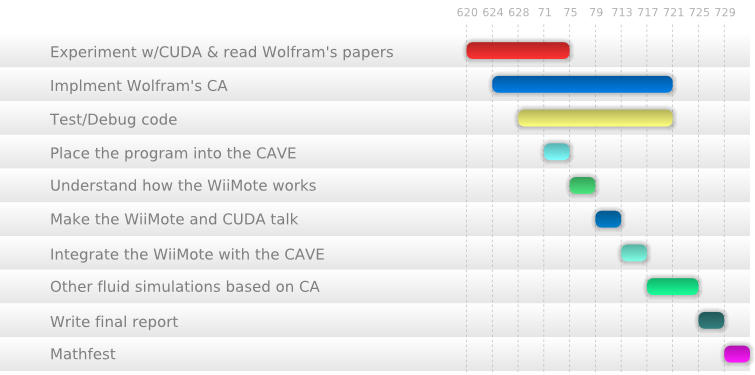# “baREUcuda” Project Proposal

Much like Stephen Wolfram, I have been fascinated by the capabilities of cellular automata (CA), and while I do not share his opinion that everything can be decomposed into a CA problem, I do believe that many problem exhibit similarities to problems in CA. This rich field is rarely explored by undergraduate students, and aside from writing basic programs that simulate the Game of Life or draw the Sierpinski triangle, I too have little intuition of the field’s application.

While the primary motivation for singling out Wolfram’s method is to speak to his intellectual interest, Wolfram’s papers can be used to explorer extensions of CA that are used for computational fluid dynamics (CFD). The reason is that a new methods of CFD such as Lattice Gas Automata (LGA) and later Lattice Boltzmann Methods (LBM) arose directly1 from 7 landmark paper on the relationship between fluid dynamics and CA by Wolfram in the latter half of the 1980s (see references).

In this project we will explore what Wolfram described in his papers, look at papers that build upon his revolutionary work, and compare them to other methods in contemporary CFD. All experiments will be simulated on CUDA enabled NVIDIA chips to maximize performance, and a 3D simulation of Naiver-Stokes equations using Wolfram’s work will be programmed on it to demonstrate and explore the parallelization of both CA and computation. Also, we will replace the conventional input devices (mice and keyboards) with the WiiMote. This would enable us to explore input methods described in current Human Interface Interaction literature. Finally, we will place our fluid simulation into the Beckman Institute’s Cube virtual environment via the cluster based distributed graphics language, Syzygy2.

# Project Timeline

The following gantt chart displays the time line for each subproject accomplished in the REU along with a brief description of what each entails.I am aware that the time line is overambitious, but I would rather be in a pinch against time than bored with nothing to do.

1. There are a few books describing CA as it relates to CFD, and Wolfram himself has written around 100 pages on the subject. It would take around a week or a week and a half to absorb the content.

Also, CUDA must be learn, and while I have written a basic matrix adder, there is more to learn in terms of optimization and interfacing with OpenGL. I thus plan on writing one 1D CA, move to 2D CA, and, finally, implement Wolfram’s ideas in 2D. Only when I have understood those will I tackle 3D.

2. Implement what I have read in the papers and optimize.
3. Optimization has never been important to me, but after programming a basic matrix adder in CUDA, I realized that I can easily cut computation by a half if I use the right directives and memory spots.
4. I will place the simulation in the Cub. This can be done by extending the existing socket connection program devised. A more interesting idea is to simulate the Mathematica kernel and use caveMathematica to display the simulation in the Cube. This method is more difficult, however.
5. I will spend some time figuring out how the Wiimote works and how to interface it with an OpenGL program first and then interface with CUDA. One potential problem, at least with the `wiiuse` library is that an infinite loop is required to capture events. I will thus examine other libraries or ways around this issue.
6. Integrate the WiiMote with the Cube. While this has been done, I would like to extend it and specifically see how it can interface with CUDA in the CAVE.
7. A few days will be reserved to examining and comparing other CFD models.
8. Write final report.
9. Prepare presentation for Mathfest, and attend the conference.

# SubProjects

• Help other REU student with their projects whenever possible.
• Write the `igl2ogl` guide.
• S. Wolfram. Minimal cellular automaton approximations to continuum systems. In Stephen Wolfram, editor, Cellular Automata and Complexity: collected papers. Addison-Wesley, 1994.
• S. Wolfram. Cellular automaton fluids 1: Basic theory. Journal of Statistical Physics, 45(3):471–526, 1986.
• S. Wolfram. Statistical mechanics of cellular automata. Reviews of Modern Physics, 55(3):601–644, 1983.
• N.H. Packard and S. Wolfram. Two-dimensional cellular automata. Journal of Statistical Physics, 38(5):901–946, 1985.
• S. Wolfram. Twenty problems in the theory of cellular automata. Physica Scripta, 9:170–183, 1985.
• S. Wolfram. Cellular automation supercomputing. 1988.
• J.B. Salem and S. Wolfram. Thermodynamics and hydrodynamics with cellular automata. Theory and Applications of Cellular Automata. World Scientific, 1986.

1. When asked on the NKS forums about the most successful application of CAs thus far, Jason Cowley, of Wolfram Research, labeled Wolfram’s work on computational fluid dynamics as the “most successful use of NKS” thus far.

2. Syzygy is a cluster-based distributed graphics system currently powering several virtual environments, such as the Cube and CAVEs at the University of Illinois, the Portal at the Technical University of Berlin, and the DiVE at Duke University.